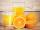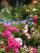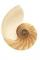# Bag of peanuts

Joe eat 1/3 of a bag of peanuts, mark eat 1/4 of the remaining in the bag of peanuts, Alvin eat 1/2 of the remaining bag of peanuts, peter eat 10 peanuts, there are 71 peanuts left. Hon many peanuts were in the bags?

Result

n =  324

#### Solution:

n/3 + 1/4 * (n-n/3) + 1/2*(n-(n/3 + 1/4 * (n-n/3))) + 10 + 71 = n

3n = 972

n = 324

Calculated by our simple equation calculator.

Leave us a comment of example and its solution (i.e. if it is still somewhat unclear...):

Showing 0 comments:Be the first to comment!#### To solve this example are needed these knowledge from mathematics:

Need help calculate sum, simplify or multiply fractions? Try our fraction calculator. Do you have a linear equation or system of equations and looking for its solution? Or do you have quadratic equation?

## Next similar examples:

1. TripOn the trip drank 3/10 of pupils tea, 2/5 cola, 1/4 mineral water and remaining 3 juice. How many students were on the trip?
2. Cleaning windowsCleaning company has to wash all the windows of the school. The first day washes one-sixth of the windows of the school, the next day three more windows than the first day and the remaining 18 windows washes on the third day. Calculate how many windows ha
3. Sales storesThe first sales store passed the 1/3 and the second 2/5 of the total amount of goods.In third store passed the 2/3 rest of the goods. The remaining 40 kg of goods put into fourth store. How many kilograms of goods pass to a third store?
4. PearsThere were pears in the basket, I took two-fifths of them, and left six in the basket. How many pears did I take?
5. RosesOn the large rosary was a third white, half red, yellow quarter and six pink. How many roses was in the rosary?
6. Equation with xSolve the following equation: 2x- (8x + 1) - (x + 2) / 5 = 9
7. Equation with fractionsSolve equation: ? It is equation with fractions.
8. Simple equation 6Solve equation with one variable: X/2+X/3+X/4=X+4
9. Unknown numberI think the number - its sixth is 3 smaller than its third.
10. EquationSolve the equation: 1/2-2/8 = 1/10; Write the result as a decimal number.
11. EqnSolve equation with fractions: 2x/3-50=40+x/4
12. UN 1If we add to an unknown number his quarter, we get 210. Identify unknown number.
13. Unknown numberIdentify unknown number which 1/5 is 40 greater than one tenth of that number.
14. Simple equationSolve for x: 3(x + 2) = x - 18
15. FractionsThree-quarters of an unknown number are 4/5. What is 5/6 of this unknown number?
16. One thirdIf 3/5 is 360, how much is 1/3?
17. Determine the numberDetermine the number x that ?.